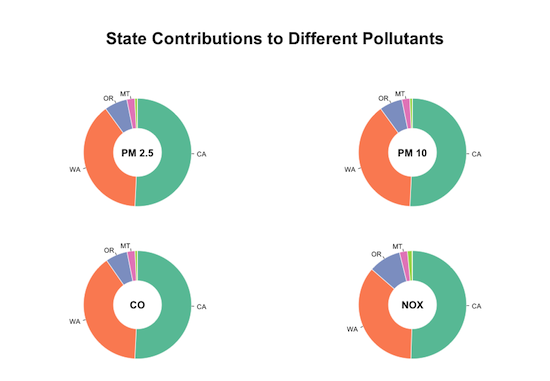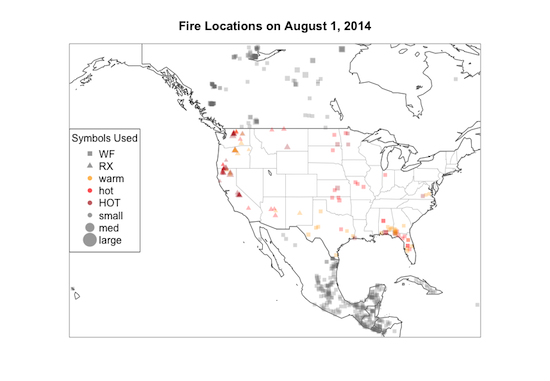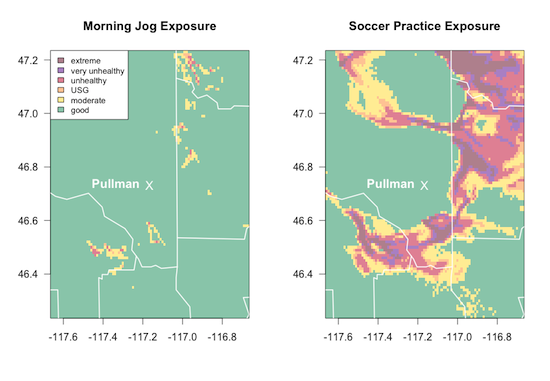The following class materials on using R were developed for the AirFire team at the USFS Pacific Wildland Fire Sciences Lab in Seattle, Washington.

The AirFire team works on monitoring and modeling wildfire emissions, smoke and air quality. Permission has been granted to release these class materials to the public in the interest of encouraging scientists in other agencies to experiment with R for their daily work. A detailed syllabus follows.

The complete class is available at this location:

http://mazamascience.com/Classes/PWFSL_2014/

Class materials are broken up into nine separate lessons that assume some experience coding but not necessarily any familiarity with R. Autodidacts new to R should take about 20-30 hrs to complete the course. The target audience for these materials consists of USFS employees or graduate students with a degree in the natural sciences and some experience using scientific software such as MATLAB or python. Lessons are presented in sequential order and assume the student already has R and RStudio set up on their computer. Additional system libraries such as NetCDF are required for later lessons.

Here is the basic outline of covered topics.

## Lesson 01 — First Steps with R

The first lesson serves as an introduction to fundamental programming concepts in R: functions, operators, vectorized data and data structures (vector, list, matrix, dataframe). By the end of the first lesson, students should be able to open and plot simple data frames and access help documents and source code associated with R functions.

## Lesson 02 — Working with Dataframes

Lesson 02 focuses on data frames and uses publicly available data on wild land fires and prescribed burns as an example. This lesson includes a discussion of factors and how to create logical masks for data subletting as well as graphical parameters used in customizing basic plots.

## Lesson 03 — ‘dplyr’ for Summary Statistics

Lesson 03 introduces the dplyr package and its core functions: filter(), select(), group_by(), summarize() and arrange(). This lesson ends with a set of tasks, encouraging students to write code similar to the following example given in the lesson:

# Take the "fires" dataset
#   then filter for type == "WF"
#   then group by state
#   then calculate total area by state
#   then arrange in descending order by total
#   finally, put the result in wildfireAreaByState
fires %>%
filter(type == "WF") %>%
group_by(state) %>%
summarize(total=sum(area, na.rm=TRUE)) %>%
arrange(desc(total)) ->
wildfireAreaByState

## Lesson 04 — Bar and Pie Plots

Lesson 04 focuses on the barplot() and pie() functions and associated plotting customizations so that students end up converting summary tables from the previous lesson into multi-panel plots:## Lesson 05 — Simple Maps

Lesson 05 introduces the maps package and uses it to plot wildfire data.## Lesson 06 — Dashboard

Lesson 06 consists of a longer script that defines several functions to encapsulate all of the work covered in previous lessons. The end result is a function that accepts a single datestamp argument, constructs an appropriate URL, imports CSV data as a data frame and then manipulates and plots that data as a summary ‘dashboard’ appropriate for use in a decision support system.

## Lesson 07 — BlueSky first Steps

Lesson 07 introduces the ncdf4 package for working with BlueSky model output predicting the spatial extent and concentration of wildfire smoke. The lesson walks through the process of reading in and understanding a NetCDF file and then presents a script to convert existing files into modernized equivalents that are easier to work with.

## Lesson 08 — Working with Arrays

The gridded model datasets introduced in Lesson 07 are made available as multi-dimensional R arrays. Lesson 08 describes in greater detail how to work with arrays and how to generate multi-dimensional statistics by using the apply() function. By the end of the lesson, students should be able to perform increasingly detailed analyses of subsets of the data.## Lesson 09 — Working with Dates and Times

Lesson 09 goes into more detail about the time dimension and covers use of the POSIXct data type and the lubridate package, especially for work involving both local and UTC timezones. The openair package is also introduced especially for the rollingMean() and timeAverage() functions which make it easier to compare time series defined on different time axes — very important when comparing model and sensor data.

We hope these lessons encourage people working in the Forest Service or other government science agencies to take a look at R and experiment with it for a variety of data management, analysis and visualization needs. R does have a steep learning curve but, once mastered, provides users with an extremely powerful and customizable tool for all sorts of analysis.

Best of Luck Learning R!